# Diode Lab

Analog Lab > Diode Lab

In analog electronics, a Diode is a component that restricts the direction of movement of Charge Carriers. It allows an Electric Current to conduct in one direction, but blocks the current in the opposite direction. One important application of Diodes is in the design of Rectifier Circuit, which is one of the important parts of a DC Power Adapter, Figure 1.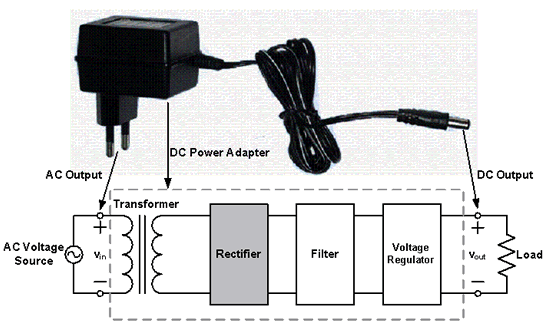Figure 1: Block Diagram of a DC Power Adapter

Rectification is the process of converting Alternating Current (AC) into Direct Current (DC). AC current and voltage change to positive and negative polarities alternately (bidirectional), while DC current and voltage only has either one polarity (unidirectional). The electrical devices used to perform rectification are called Rectifiers. Rectification is classified as Half-wave or Full-wave, with Half-wave being the simplest.

Half-wave Rectifiers allow either the positive or negative half cycles of the AC to pass and block the other one. It can be implemented by a Diode. An example of a positive Half-wave Rectifier is given in Figure 2a. The Diode conducts during positive half cycles, therefore it can be treated as a short circuit, Figure 2b. On the other hand, it blocks the current during the negative half cycles, therefore it can be treated as an open circuit, Figure 2c.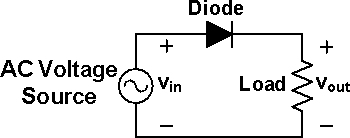Figure 2a: A Diode acts as a Half-wave Rectifier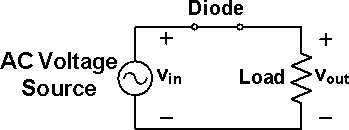Figure 2b: The Diode is equivalent to a short circuit during positive half cycles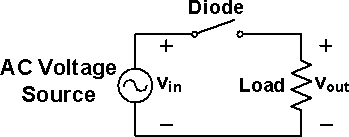Figure 2c: The Diode is equivalent to an open circuit during negative half cycles

The input and output voltage Waveforms of a Positive Half-wave Rectifier are shown in Figure 3a and Figure 3b respectively. The output is unidirectional and has a finite average value, DC component.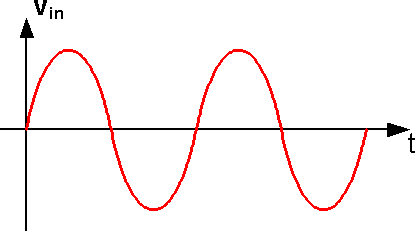Figure 3a: AC Input Voltage Waveform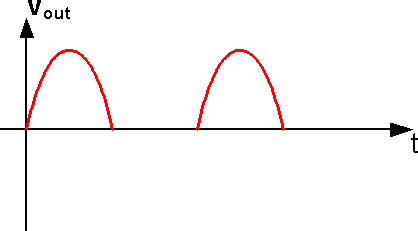Figure 3b: DC Output Voltage Waveform after Positive Half-wave Rectifier

Full-wave Rectifiers allow both half cycles of AC to pass. Figure 4a is the circuit of a Full-wave Bridge Rectifier, it is constructed by four Diodes, during each half cycle only 2 out of 4 Diodes are conducting, Figure 4b and Figure 4c.

During positive half cycles, as shown in Figure 4b, current is conducted through D4, the load Resistor and D6. Meanwhile, D3 and D5 are reverse-biased, thus no current flows through them. During negative half cycles, as shown in Figure 4c, current flows through D3, the load Resistor and D5. Meanwhile, D4 and D6 are reverse-biased.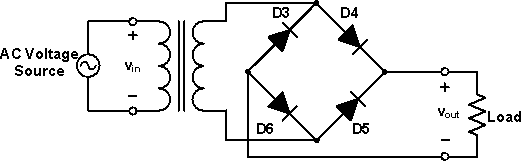Figure 4a: Full-wave Rectifier circuit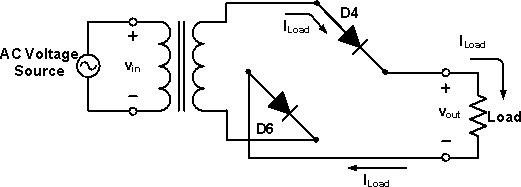Figure 4b: Equivalent rectifier circuit during the positive half cycles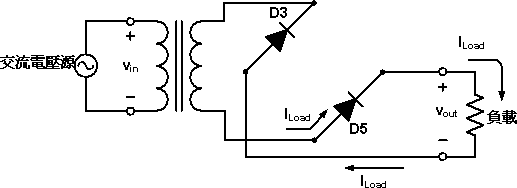Figure 4c: Equivalent rectifier circuit during the negative half cycles

During both half cycles, current flows through the load resistor in the same direction (from up to down). The voltage across the load resistor is always positive. The input and output voltage Waveforms of a Full-wave Rectifier are shown in Figure 5a and Figure 5b respectively. The Full-wave Rectifier utilizes both half cycles of the input, which makes it the most popular rectifier configuration.Figure 5a: Input AC Voltage Waveform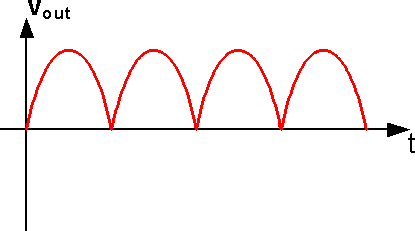Figure 5b: Output DC Voltage Waveform after Full-wave Rectification

If a Capacitor is added in parallel with the load resistor of a Rectifier to form a simple Filter Circuit, the output of the Rectifier will be transformed into a more stable DC Voltage, Figure 6. At first, the capacitor is charged to the peak value of the rectified Waveform. Beyond the peak, the capacitor is discharged through the load resistor until the time at which the rectified voltage exceeds the capacitor voltage. Then the capacitor is charged again and the process repeats itself.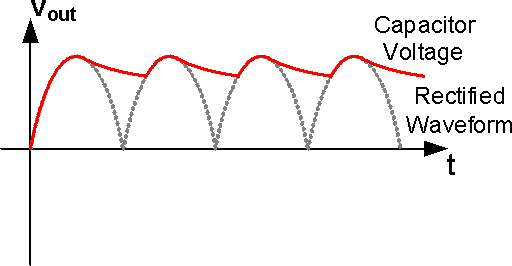Figure 6: Output Voltage Waveform (solid line) of Full-wave Rectifier with Filter Capacitor added

Analog Lab > Diode Lab